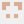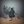0%
0%

# Object Following Control of a Robotic Camera Arm

Virtual Reality, Kalman Filter, and Inverse Dynamics Control

Similar projects worth following
3.9k views
This is a 5 DOF robotic camera arm that is capable of tracking an object while following a given trajectory. A virtual reality software package is used to generate the trajectory. The system currently exploits "record and play" operation principle. A motion control algorithm is developed and simulated in the MapleSim environment. An improved version is engineered which slides on a rail system while tracking the object. The simulation results are provided in the project logs. Fusion of the different sources of sensor data (gyroscope and accelerometer) is done by a Kalman Filter.

The potential use of this product is demonstrated by the following video where the camera tracks the motion, orbits around the point of interest and rotates about itself, creating an extraordinary footage.

The simulation of the product is as follows:

Note that the blue arrow shows the direction at which the camera is pointing. Also, the trajectory that is given in red, can be altered as desired using the Virtual Reality add-on.

1. Virtual Reality is an optional step for producing the intermediate positions in the camera trajectory.
2. Trajectory generation: Given start, end, and intermediate positions, the quantic trajectory polynomial generates the end-effector trajectory in MATLAB.
3. These Cartesian coordinates are exported to the robot manipulator simulation.
4. If the simulation works as expected, the trajectory is transmitted to the microcontroller via serial communication.
5. The camera orientation can be controlled by a human operator or by the object detection algorithm.
6. The position feedback is transferred into the LabVIEW environment and used for monitoring the data and simulating the results on the 3D model.

The circuitry is completed. An image is provided below.

### Robo1.csv

The .csv file exported from the Simulation

ms-excel - 47.87 kB - 08/13/2017 at 21:55

### Servo_Calibration.zip

Code to test the Robot Arm

x-zip-compressed - 6.25 kB - 08/13/2017 at 21:55

### 1874637.pdf

Data Sheet Step-down converters

Adobe Portable Document Format - 491.06 kB - 05/12/2017 at 14:23

### Data Sheet Servo Motor.pdf

Adobe Portable Document Format - 349.49 kB - 05/08/2017 at 19:59

• 6 × HJ S3315D Metal Gear Servo Motor - Torque: 15 kgcm
• 1 × Micro servo Torque: 1.6 kgcm
• 2 × NUCLEO-F401RE STM32 Microcontroller
• 1 × Raspberry Pi Camera
• 1 × Arduino Bluetooth Shield
• ### Assembly Completed

Canberk Suat Gurel08/18/2017 at 11:10 0 comments

The robot arm assembly is completed and the circuitry is tested.

The figure below shows the rest position of the robot arm. It can be seen that the Servo 4 has a slight offset of about 45 degrees. This issue can easily be fixed as shown in the 2nd figure.

• ### MATLAB and Joystick

Canberk Suat Gurel08/13/2017 at 22:25 0 comments

As of know, the robot arm is fully functional and tested with the Serve Calibration code.

I have initially written the code on Arduino Web Editor but then installed an add-on to MATLAB and started using MATLAB to program my microcontroller. This is a huge advantage since all the computation is done on MATLAB (instead of the microcontroller) and MATLAB has a greater memory compared to the microcontroller.

Know we can control the robot arm (live time) using inverse kinematics. As the diagram in the appendix shows, the coordinate data will be inputted from a Joystick. The video below shows a quick demo.

• ### Inverse Dynamics Controller

Canberk Suat Gurel06/16/2017 at 13:58 0 comments

Inverse dynamics is a nonlinear control technique that provides a better trajectory tracking by calculating the required joint actuator torques that need to be generated by the motors to achieve a given trajectory.

The matrix form of the dynamics of a robot leg is given by Equation 44 where the and terms are neglected.

For a given and the non-linearity of the system can be vanished, making the system linear and hence the exact amount of torque that needs to be generated by the joint actuator to overcome the inertia of the actuator can be calculated. If the and match with the desired and then the projected trajectory will be tracked as expected .

Equation 45 shows the control law expression where is a new input to the system. The numeric value of is calculated by Equation 46 where is the desired trajectory, is the actual trajectory, and and are position and velocity gains, respectively .

Since the mass matrix, M, is invertible, equating Equation 45 to Equation 46 gives the expression in Equation 47 which shows that the new system is linear as it is given by Equation 48.

Figure 4.30 demonstrates the inner loop and outer loop control architecture where the output of the system yields the joint space signal which enables a better trajectory tracking given that the feedback gains and are tuned as appropriate.

• ### Dynamic Model of Mechanical Drive Systems

Canberk Suat Gurel06/16/2017 at 13:45 0 comments

In the MapleSim environment, the rotations of the revolute joints are controlled in joint space where are altered directly by applying signals to the joints. However, in real life, the rotation of the joint actuator is controlled by control signals, i.e. voltage or current. Therefore, the dynamic model of the motion system is crucial to deduce the relationship between the control signals and the rotation of the revolute joints. It is also used to obtain the relationship between control signal and joint actuator forces which serves the purpose of choosing the suitable actuators. The Figure 1 shows the mechanical model of a permanent magnet (PM) DC motor that is connected to an inertial load through a gearbox with a ratio of.

Figure 1

The fundamental mechanical relationship that defines the system in Figure 3 is given by Equation 1 which is also referred as torque balance equation

where the terms are given by Table 1.

Table 1

• ### Test Trajectory

Canberk Suat Gurel06/15/2017 at 09:50 0 comments

The image below shows the trajectory that is used for testing the trajectory tracing of the robot manipulator. The Cartesian coordinates will be converted into joint rotations using the derived inverse kinematic equations, then these angles (CSV file) will be read using the Processing language.

The individual joint profiles are as follows,

• ### Testing the Motors

Canberk Suat Gurel05/27/2017 at 18:56 0 comments

It can be seen in the video that the servo motors are configured and working. There is an undesirable vibration during the operation, that is because of using a potentiometer to control the angle of rotation. In reality, this will be done by a quintic trajectory planner which ensures a smooth motion when it is combined with a inverse dynamic controller. I will talk more about this in the following logs.

• ### 3D Design

Canberk Suat Gurel05/13/2017 at 22:39 0 comments

The following visuals belong to the initial design.

• ### VR for Trajectory Generation

Canberk Suat Gurel05/08/2017 at 20:16 0 comments

The trajectory is generated on record and play basis. This is achieved by utilizing an open-source virtual reality software. An example is provided below.

• ### Kinematics

Canberk Suat Gurel05/07/2017 at 14:53 0 comments

Robot manipulator kinematics

The image below shows the incomplete assemble of the robot manipulator.

• ### Voltage Regulation Circuitry

Canberk Suat Gurel05/07/2017 at 14:31 0 comments

Servo motors - as you can see from the datasheet provided in the Files - require 7.4V to operate at maximum torque of 16kgcm. However our experiments showed that motor windings got damaged due to high heat that had been generated. For this reason, I decided to supply 6V to each motor. The PCB on the left, consists of 2 step down converters. It supplies variable voltage that can be adjusted anywhere between 20V to 3V and maximum 6A. The PCB on the right is the connection to the motors.

The circuits given above are supplied by a computer power supply which supplies 12V to each of the 4 step down converters. The datasheet of the converters are provided in the "files" section. The image below shows the computer power supply.

The following YouTube video is a tutorial that shows how it should be activated and connected to the rest of the circuitry.

Share

## Discussions

Kristen Swanner wrote 05/20/2019 at 17:55 point

Thank you for sharing your work! You've done a great job!

Are you sure? yes | no

## Similar Projects

Project Owner Contributor

### Design of Autonomous Logistics Transportation Robossla-couk

Project Owner Contributor

### Autonomous Logistics Transportation Robotensafatef

# Does this project spark your interest?

Become a member to follow this project and never miss any updates